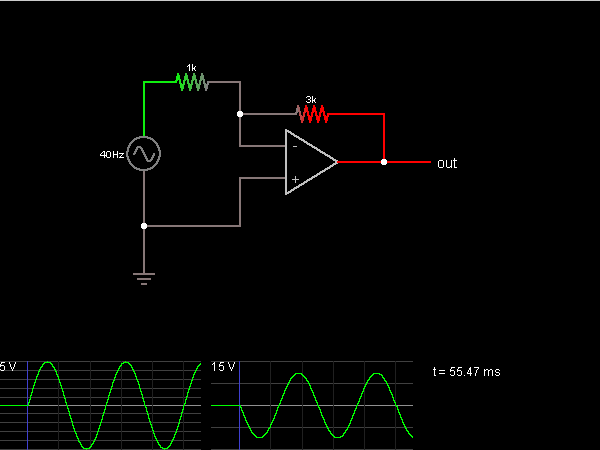# Circuit Simulator - Inverting Amplifier

## Where can I get Inverting Amplifier Circuit Diagram with Explanation?

IndiaBIX provides you lots of fully solved Inverting Amplifier circuit diagram with detailed explanation and working principles.

## How to design a Inverting Amplifier (electronic circuit)?

You can easily design the Inverting Amplifier circuit by practicing the exercises given below. Here you can design and simulate your own electronic circuits with this Online Circuit Designer and Simulator.### Circuit Description:

This circuit inverts and amplifies the input, multiplying the voltage by -3, using an op-amp. When connected in a negative feedback configuration, the op-amp attempts to keep its two inputs at the same voltage. One is at ground, so for the other one to be at ground, there must be a voltage drop across the 1k resistor equal to the input voltage. The 3k resistor has the same current across it, so the voltage drop must be 3 times as large, by Ohm's Law, making the output equal to -3 times the input voltage. -- Credits: Mr. Paul Falstad.